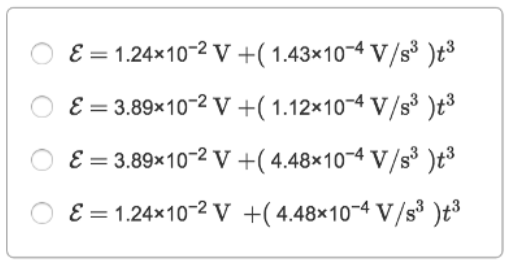# Problem: A coil 4.50 cm radius, containing 510 turns, is placed in a uniform magnetic field that varies with time according to B = (1.20x10-2 T/s) t + (3.45x10-5 T/s4)t4. The coil is connected to a 650-Ω resistor, and its plane is perpendicular to the magnetic field. You can ignore the resistance of the coil.Part AFind the magnitude of the induced emf in the coil as a function of time.Part BWhat is the current in the resistor at time t0 = 4.75 s?

###### FREE Expert Solution

A.

Induced emf:

$\overline{){\mathbit{\epsilon }}{\mathbf{=}}{\mathbit{N}}{\mathbit{A}}\frac{\mathbf{dB}}{\mathbf{dt}}}$

Power rule of derivation:

$\overline{)\frac{\mathbit{d}}{\mathbit{d}\mathbit{t}}\mathbf{\left(}{\mathbit{x}}^{\mathbit{n}}\mathbf{\right)}{\mathbf{=}}{\mathbit{n}}{{\mathbit{x}}}^{\mathbit{n}\mathbf{-}\mathbf{1}}}$

A = πr2

87% (230 ratings)###### Problem Details

A coil 4.50 cm radius, containing 510 turns, is placed in a uniform magnetic field that varies with time according to B = (1.20x10-2 T/s) t + (3.45x10-5 T/s4)t4. The coil is connected to a 650-Ω resistor, and its plane is perpendicular to the magnetic field. You can ignore the resistance of the coil.

Part A
Find the magnitude of the induced emf in the coil as a function of time.Part B

What is the current in the resistor at time t0 = 4.75 s?